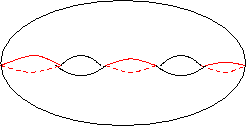#### Higher Genus Curves

We have seen how to construct spheres and tori along with various singular objects. We now want to consider curves with large genus (i.e. many holes). A genus two surface or "two-holed doughnut" is depicted below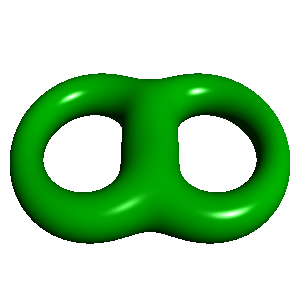The basic idea would be to use higher degree equations. Consider the quartic equation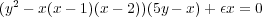For small nonzero ε this is gives the nonsingular curve graphed in red. However, when ε =0, this is a reducible curve which is a union of a nonsingular cubic (blue) and a line (green).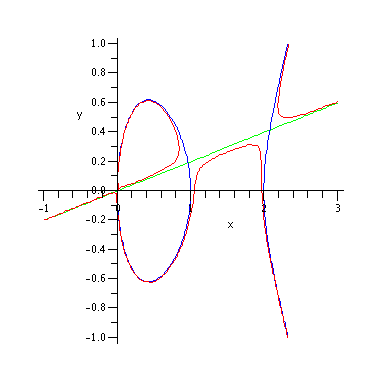When the points of infinity are added, we get a line meeting the cubic in 3 points. The complex picture of this reducible curve looks something like this: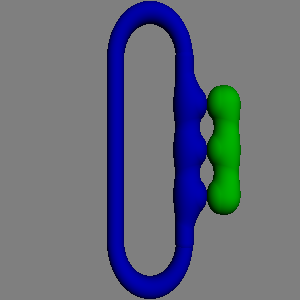As ε changes to nonzero values, the points of contact "open up" into tubes resulting in a genus 3 curve.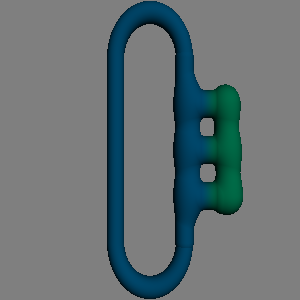In general if g(d) is the genus of a degree d nonsingular curve, then proceeding as above, we obtain a nonsingular genus d +1 curve by "smoothing" the union of a genus d curve and a line. The line meets the degree d curve in d points, so this leads to an inductive formula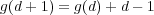from which we get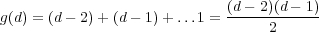We have been a bit vague about what it means to add points at infinity. The real answer is that we do this by working in the projective plane, and then resolving singularities at infinity if necessary. The above formula only applies when there are no singularities including at infinity. If it is necessary to resolve singularities, then the genus of the new curve will be less than what the formula predicts.

To get a genus 2 example, we can instead consider a hyperelliptic curve defined by an equation y2 = p(x) where p(x) is a polynomial of degree with at least 5 distinct roots. The last condition guarantees it's nonsingular in C2 although it will have singularities at infinity. In particular, the above genus formula doesn't apply, but it can be computed by Riemann-Hurwtiz formula given in the next section. The curve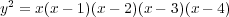would have genus 2. This is partly evident from the real graph: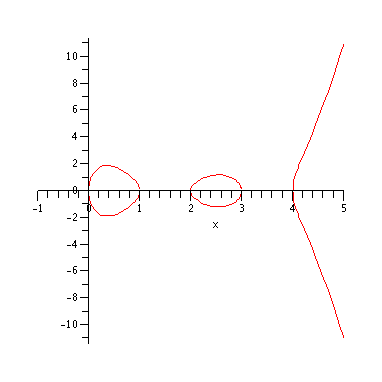which correspond to the red curves marked on the complex picture